## How to Build a Parameterized Archimedean Spiral Geometry

#####Kateryna Vyshenska July 27, 2016

Archimedean spirals are often used in the analysis of inductor coils, spiral heat exchangers, and microfluidic devices. Today, we will demonstrate how to build an Archimedean spiral using analytic equations and their derivatives to define a set of spiral curves. Based on these curves, we will then create a 2D geometry with specific thickness, extruding it to a full 3D geometry.

### A Brief Introduction to Archimedean Spirals

Widely observed in nature, spirals, or helices, are utilized in many engineering designs. As an electrical engineer, for instance, you may wind inductive coils in spiral patterns and design helical antennas. As a mechanical engineer, you may use spirals when designing springs, helical gears, or even the watch mechanism highlighted below.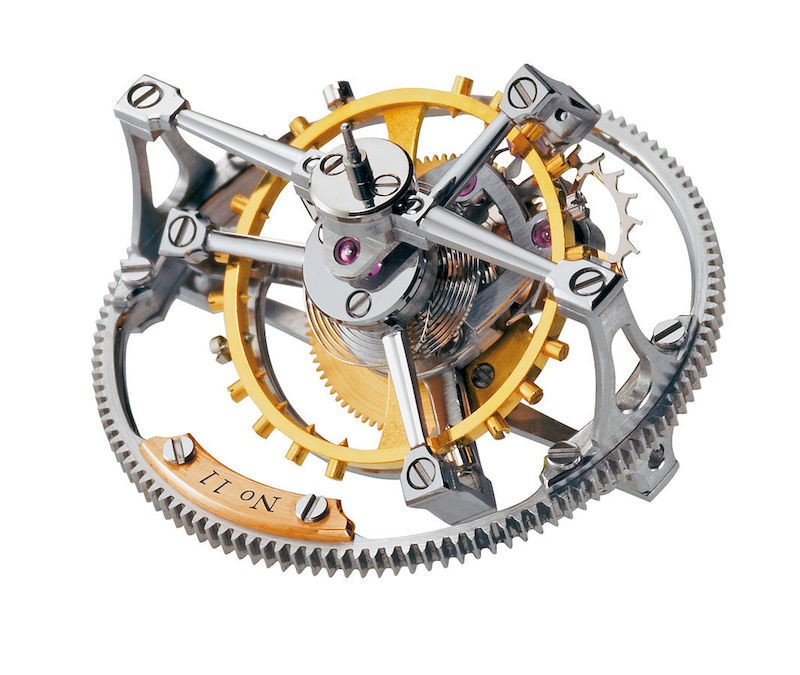An example of an Archimedean spiral used in a clock mechanism. Image by Greubel Forsey. Licensed by CC BY-SA 3.0, via Wikimedia Commons.

Here, we’ll focus on a specific type of spiral, the one that is featured in the mechanism shown above: an Archimedean spiral. An Archimedean spiral is a type of a spiral that has a fixed distance between its successive turns. This property enables it to be widely used in the design of flat coils and springs.

We can describe an Archimedean spiral with the following equation in polar coordinates:

r=a+b\theta

where a and b are parameters that define the initial radius of the spiral and the distance between its successive turns, the latter of which is equal to 2 \pi b. Note that an Archimedean spiral is also sometimes referred to as an arithmetic spiral. This name derives from the arithmetic progression of the distance from the origin to point on the same radial.

### Designing a Parameterized Archimedean Spiral Geometry

Now that we’ve introduced Archimedean spirals, let’s take a look at how to parameterize and create such a design for analysis in COMSOL Multiphysics.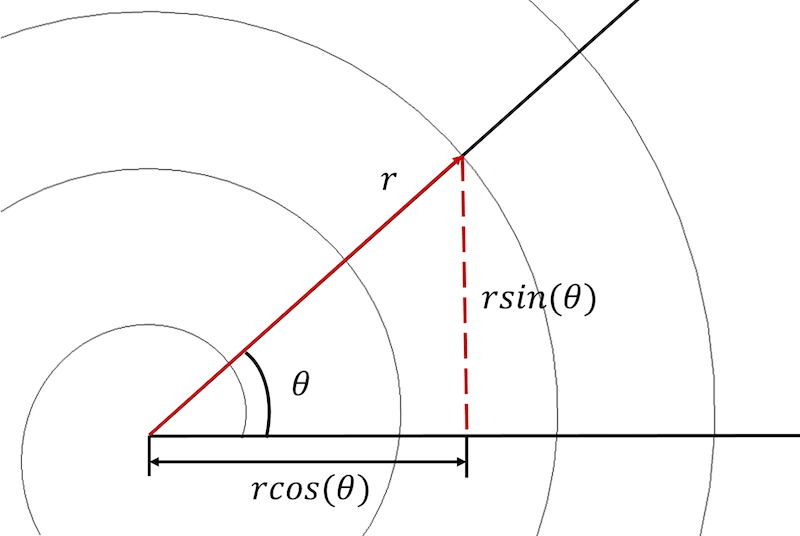An Archimedean spiral can be described in both polar and Cartesian coordinates.

To begin, we need to convert the spiral equations from a polar to a Cartesian coordinate system and express each equation in a parametric form:

\begin{align*}
x_{component}=rcos(\theta) \\
y_{component}=rsin(\theta)
\end{align*}

This transformation allows us to rewrite the Archimedean spiral’s equation in a parametric form in the Cartesian coordinate system:

\begin{align*}
x_{component}=(a+b\theta)cos(\theta) \\
y_{component}=(a+b\theta)sin(\theta)
\end{align*}

In COMSOL Multiphysics, it is necessary to decide on the set of parameters that will define the spiral geometry. These parameters are the spiral’s initial radius a_{initial}, the spiral’s final radius a_{final}, and the desired number of turns n. The spiral growth rate b can then be expressed as:

b=\frac{a_{final}-a_{initial}}{2 \pi n}

Further, we need to decide on the spiral’s start angle theta_0 and end angle theta_f. Let’s begin with the values of theta_0=0 and theta_f=2 \pi n. With this information, we are able to define a set of parameters for the spiral geometry.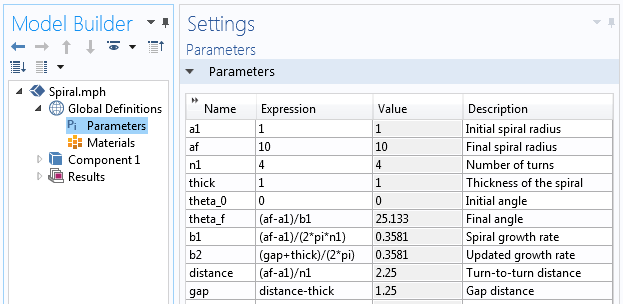The parameters used to build the spiral geometry.

To build this spiral, we’ll start with a 3D Component and create a Work Plane in the Geometry branch. In the Work Plane geometry, we then add a Parametric Curve and use the parametric equations referenced above with a varying angle to draw a 2D version of the Archimedean spiral. These equations can be directly entered into the parametric curve’s Expression field, or we can first define each equation in a new Analytic function as:

\begin{align*}
X_{fun}=(a+bs)cos(s) \\
Y_{fun}=(a+bs)sin(s) \\
\end{align*}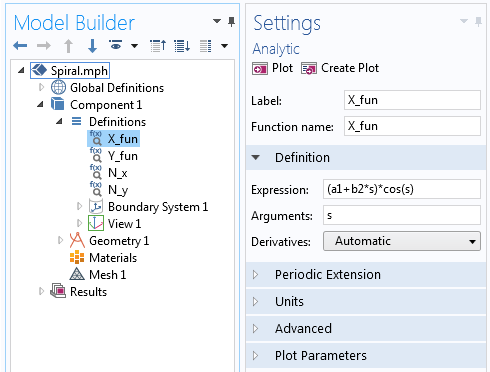The X-component of the Archimedean spiral equation defined in the Analytic function.

The Analytic function can be used in the expressions for the Parametric Curve. In this Parametric Curve, we vary parameter s from the initial angle of the spiral, theta_0, to the final angle of the spiral, theta_f=2 \pi n.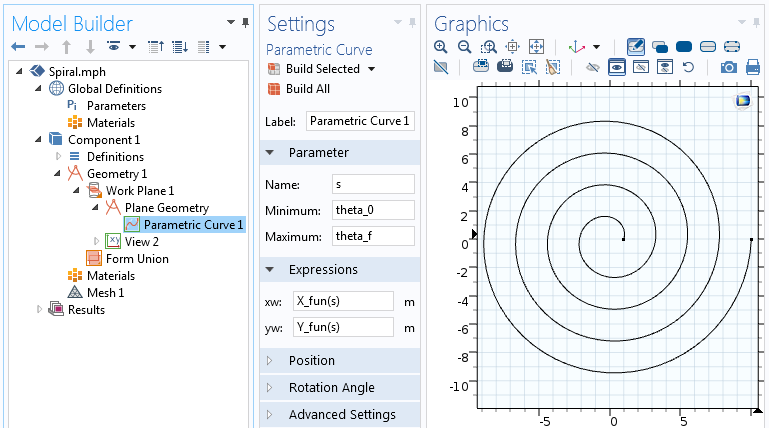The settings for the Parametric Curve feature.

The parametric spiral equations used in the Parametric Curve feature will result in a spiral represented by a curve. Let’s now build upon this geometry, adding thickness to it in order to create a 2D solid object.

Up to this point, our spiral has been parameterized in terms of the initial radius a_{initial}, final radius a_{final}, and desired number of turns n. Now, we must incorporate thickness as another control parameter in the spiral equation.

Let’s begin with the main property of the spiral, which states that the distance between the spiral turns is equal to 2 \pi b. This is also equivalent to \frac{a_{final}-a_{initial}}{n}. To incorporate thickness, we represent the distance between each successive turn of the spiral as a sum of the spiral thickness and the remaining gap between turns, thick+gap.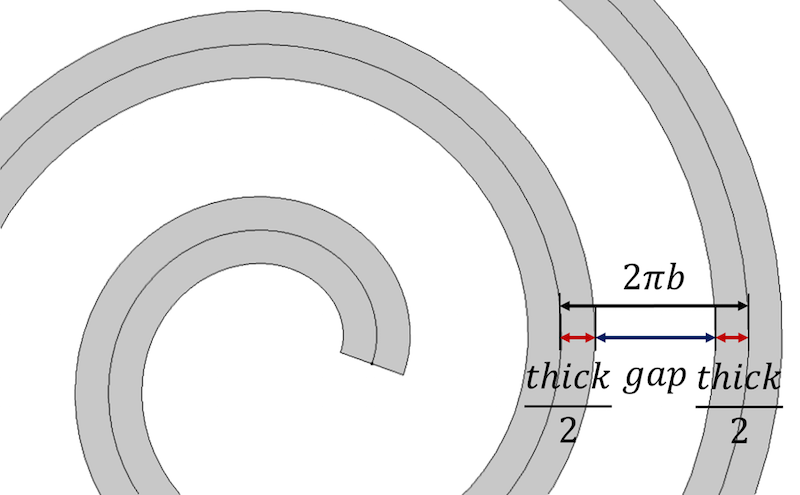The distance between spirals turns is defined in terms of the spiral thickness and gap parameters.

To control thickness and obtain identical distance between the turns, the distance can be expressed as:

\begin{align*}
distance=\frac{a_{initial}-a_{final}}{n} \\
gap=distance-thick
\end{align*}

After defining thickness and expressing the gap between turns in terms of thickness and constant distance between centerlines of the spiral, we can rewrite the spiral growth parameter in terms of thickness as:

\begin{align*}
distance=2\pi b \\
b=\frac{gap+thick}{2\pi}
\end{align*}

We will also want to express the final angle of the spiral in terms of its initial and final radii:

\begin{align*}
\theta_{final}=2 \pi n \\
a_{final}=\text{total distance}+a_{initial} \\
a_{final}=2 \pi bn+a_{initial} \\
n=\frac{a_{final}-a_{initial}}{2 \pi b} \\
\theta_{final}=\frac{2 \pi (a_{final}-a_{initial})}{2 \pi b} \\
\theta_{final}=\frac{a_{final}-a_{initial}}{b}
\end{align*}

Want to start the spiral from an angle other than zero? If so, you will need to add this initial angle to your final angle in the expression for the parameter: theta_f=\frac{a_{final}-a_{initial}}{b}+theta_0.

Duplicating the existing spiral curve twice and placing these curves with an offset of -\frac{thick}{2} and +\frac{thick}{2} with respect to the initial spiral curve allows us to build the spiral with thickness. To position the upper and lower spirals correctly, we must make sure that the offset spirals are normal to the initial spiral curve. This can be achieved by multiplying the offset distance \pm\frac{thick}{2} by the unit vector normal to the spiral curve. The equations of the normal vectors to a curve in parametric form are:

where s is the parameter used in the Parametric Curve feature. To get a unit normal, we need to divide these expressions by the length of the normal:

\sqrt{(dx/ds)^2+(dy/ds)^2 }

Our updated parametric equations for the Archimedean spiral with a half-thickness shift are:

\begin{align*}
x_{component}=(a+bs)cos(s)-\frac{dy/ds}{\sqrt{(dx/ds)^2+(dy/ds)^2}}\frac{thick}{2} \\
y_{component}=(a+bs)sin(s)+\frac{dx/ds}{\sqrt{(dx/ds)^2+(dy/ds)^2}}\frac{thick}{2}
\end{align*}

Writing out these equations in the parametric curve’s expression fields can be rather time consuming. As such, we introduce the following notation:

\begin{align*}
N_x=-\frac{dy/ds}{\sqrt{(dx/ds)^2+(dy/ds)^2}} \\
N_y=\frac{dx/ds}{\sqrt{(dx/ds)^2+(dy/ds)^2 }}
\end{align*}

where each N_x and N_y is defined via the Analytic function in COMSOL Multiphysics, similar to how we defined X_{fun} and Y_{fun} for the first parametric curve. Within the function, we use the differentiation operator, d(f(x),x), to take the derivative, as depicted in the following screenshot.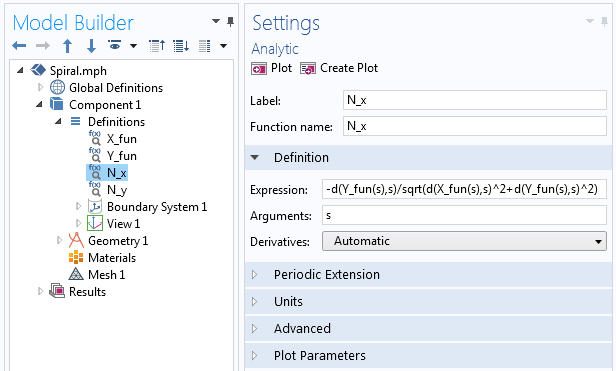Examples of the derivative operator used in the Analytic function.

The functions X_{fun}, Y_{fun}, N_x, and N_y can then be used directly in the parametric curve’s expressions for the curve on one side:

\begin{align*}
x_{lower}=X_{fun}(s)+N_x(s)\frac{thick}{2} \\
y_{lower}=Y_{fun}(s)+N_y(s)\frac{thick}{2}
\end{align*}

The functions can also be used for the curve on the other side:

\begin{align*}
x_{upper}=X_{fun}(s)-N_x(s)\frac{thick}{2} \\
y_{upper}=Y_{fun}(s)-N_y(s)\frac{thick}{2}
\end{align*}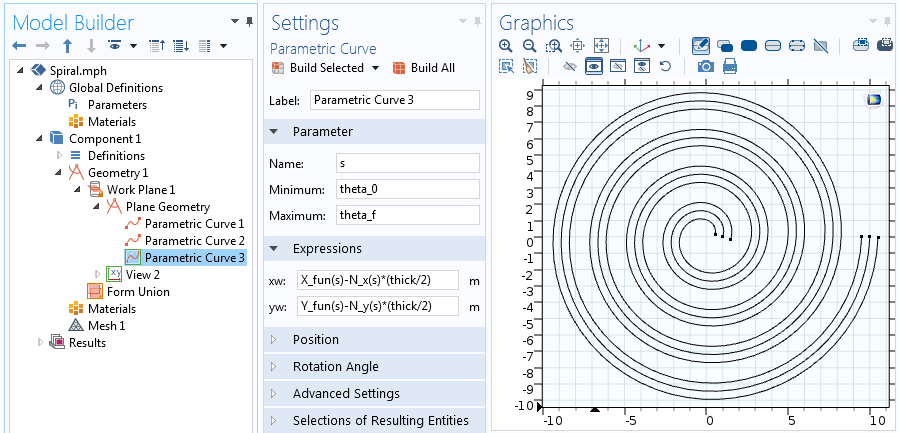Equations for the second of the two offset parametric curves.

To join the ends of two curves, we add two more parametric curves using a slight modification of the equations mentioned above. For the curve that joins the center of the spiral, we have to evaluate X_{fun}, Y_{fun}, N_x, and N_y for the starting value of the angle, theta. For the curve that joins the outer side of the spiral, we have to evaluate the final value of theta. Therefore, the joining curve in the center is:

\begin{align*}
X_{fun}(theta_0)+s\cdot N_x(theta_0)\cdot\frac{thick}{2} \\
Y_{fun}(theta_0)+s\cdot N_y(theta_0)\cdot\frac{thick}{2}
\end{align*}

The outer joining curve, meanwhile, is:

\begin{align*}
X_{fun}(theta_f)+s\cdot N_x(theta_f)\cdot\frac{thick}{2} \\
Y_{fun}(theta_f)+s\cdot N_y(theta_f)\cdot\frac{thick}{2}
\end{align*}

In both of the above equations, s goes from -1 to +1, as shown in the screenshot below.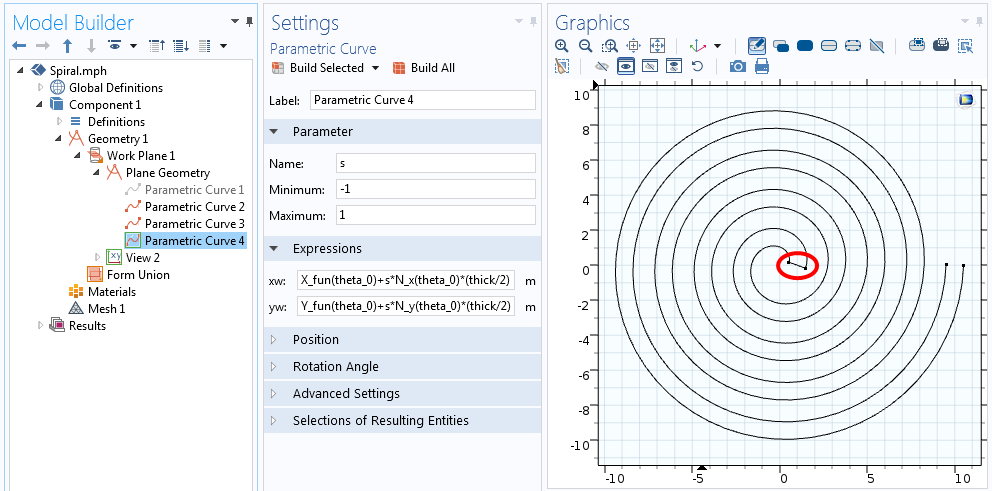Equations for the curve that joins one end of the spiral.

We now have five curves that define the centerline of the spiral and all four sides of the profile. We can disable (or even delete) the curve describing the centerline since it isn’t truly necessary, leaving just the spiral outline. With the outline of our spiral defined, the Convert to Solid operation can be used to create a single geometry object. This 2D spiral can finally be extruded into 3D via the Extrude operation.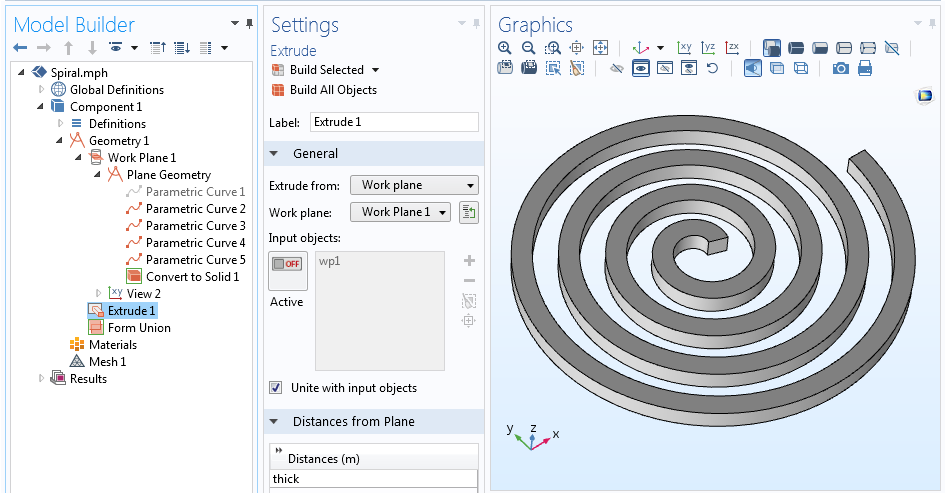The full geometry sequence and extruded 3D spiral geometry.

### Closing Remarks on Modeling Archimedean Spirals in COMSOL Multiphysics

We have walked you through the steps of creating a fully parameterized Archimedean spiral. With this spiral geometry, you can change any of the parameters and experiment with different designs, or even use them as parameters in an optimization study. We encourage you to utilize this technique in your own modeling processes, advancing the analysis of your particular spiral-based engineering design.

### Further Resources on Designing and Analyzing Spirals

• To explore further applications of simulation in the design of spiral models, try out these tutorial models:
• Read a related user story: “Analysis of Spiral Resonator Filters

#### Post Tags

Technical Content

1. Ahmed AYASH August 17, 2016   2:05 pm

Many thanks…..really is very informative blog

2. Verónica Rodríguez June 1, 2017   5:34 pm

Thank you!
Is very explicit

3. Nazım Can Öner July 15, 2019   8:11 am

Thank you for your efforts, it helped a lot.
I have got a problem. When I extrude, it doesn’t appear like a solid part. Only my curves gain height…